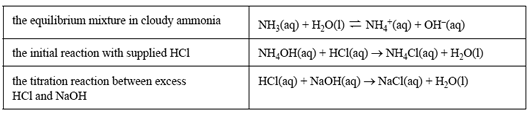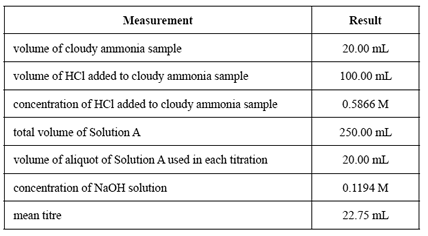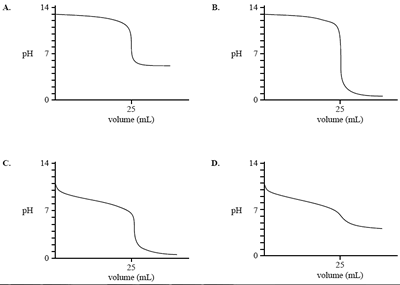Volumetric analysis (2015 VCE) 1)A small group of Chemistry students analysed household cloudy ammonia (a detergent used in domestic cleaning). A back titration was used because the detergent contained ammonia, which is very volatile. The teacher's instructions for the analysis were as follows: Step 1 – Pipette 20.00 mL of the cloudy ammonia into a 250.00 mL volumetric flask. Step 2 – Add 100.00 mL of hydrochloric acid, which is in excess. Step 3 – Make the volume up to the 250 mL mark with deionised water. Label this 'Solution A'. Step 4 – Fill a burette with sodium hydroxide solution. Step 5 – Transfer a 20.00 mL aliquot of Solution A (from Step 3) to a titration flask. Add indicator and titrate with the sodium hydroxide solution. Step 6 – Repeat Step 5 until three concordant results are obtained. The relevant equations for this analysis are as follows.The students' results for the analysis are shown in the table below.a) Calculate the amount, in moles, of hydrochloric acid initially added to the undiluted ammonia sample Solutionb) Calculate the amount, in moles, of excess hydrochloric acid in a 20.00 mL aliquot of the diluted solution from Step 5. SolutionThe manufacturer claims that the detergent contains 45.2 g L–1 ammonia as ammonium hydroxide, NH4OH. c) Use the students' experimental results to calculate: - the amount, in moles, of HCl that reacted with the ammonia in the titration flask - the amount, in moles, of ammonia initially pipetted into the 250 mL volumetric flask - the concentration, in g L–1 , of NH4OH in the cloudy ammonia sample Solution d) Provide one explanation for any difference between the students' results and the manufacturer's claim. Solution 2) Which one of the following graphs represents the pH change when a weak acid is added to a strong base?Solution